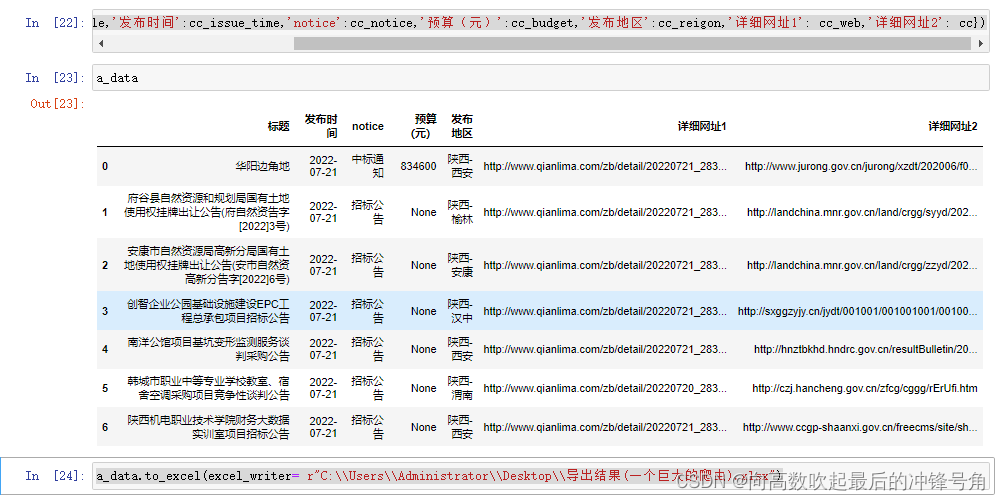# pandas存储excel方法

``````a_data=pd.DataFrame()#你的数据集

a_data.to_excel(excel_writer= r"#你想要存储的路径\\你想要存储的文件名.xlsx")``````

``````a_data=pd.DataFrame({'标题':cc_title,'发布时间':cc_issue_time,'notice':cc_notice,'预算（元）':cc_budget,'发布地区':cc_reigon,'详细网址1': cc_web,'详细网址2': cc})

``````#可以这样写
#或者这样写其实除了pandas库可以处理excel存储的问题外

``````import xlwt
workbook=xlwt.Workbook(encoding='utf-8')

DATA=((数据标签的位置),

(第一行数据),

(第二行数据),

#以此类推

)

for i,row in enumerate(DATA):
for j,col in enumerate(row):

booksheet.write(i,j,col)

workbook.save('你想存储的文件名.xls')

#默认存储在Desktop中``````

pandas除了excel储存与读取

``````#举例说明
pd.DataFrame() # 自己创建数据框，用于练习

``````